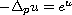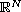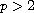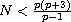Electron. J. Differential Equations, Vol. 2016 (2016), No. 326, pp. 1-5.

### Nonexistence of stable solutions to p-Laplace equations with exponential nonlinearities Phuong Le

Abstract:
In this note we prove the nonexistence of stable solutions to the p-Laplace equationon the entire Euclidean space, whereand.

Submitted November 3, 2016. Published December 22, 2016.
Math Subject Classifications: 35A01, 35B06, 35B35, 35J92.
Key Words: p-Laplace equations; stable solutions; exponential nonlinearity; nonexistence.

Show me the PDF file (185 KB), TEX file for this article.Phuong Le Department of Economic Mathematics Banking University of Ho Chi Minh City Vietnam email: phuongl@buh.edu.vn